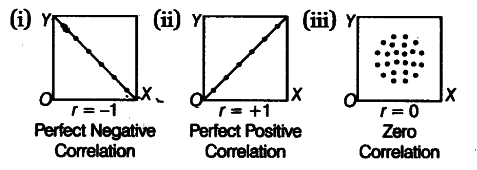# Draw three hypothetical scatter diagrams

Draw three hypothetical scatter diagrams showing the following value of r.
(i)r = -1
(ii)r =+1
(iii)r=0